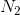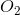GRE Subject Test: Chemistry : Gas Laws

Example Questions

Example Question #251 : Gre Subject Test: Chemistry

A gas sample is contained in a 4L vessel at a pressure of 3atm. Assuming all other conditions are kept constant, what is the new pressure in the vessel if the volume is reduced to 1.5L?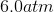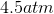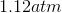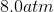Explanation:

According to Boyle's law, pressure and volume are inversely proprotional to each other. This is represented by the equation: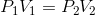In other words, as volume decreases in a vessel, the pressure will increase, and vice versa. Using the given conditions, we can solve for the final pressure in the vessel: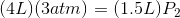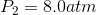Example Question #21 : Physical Chemistry

An unknown amount of neon gas is contained in a 3.00L vessel. At a temperature of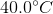, the gas exerts a pressure of 4.00atm.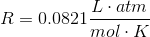Neon gas has a molar mass of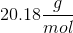.

Based on these conditions, what is the mass of neon gas in the vessel?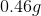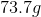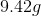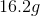Explanation:

This question deals with the amount of gas present in a vessel for only one set of conditions. This makes the ideal gas law a suitable equation to use in order to determine the amount of gas in the vessel. The ideal gas law is written as: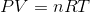Using this equation, we can solve for the molar quantity of gas in the vessel: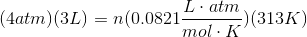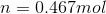Knowing this, we can now solve for the mass of the gas in the vessel by multiplying this molar amount by the molar mass: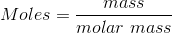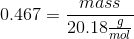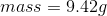Example Question #21 : Physical Chemistry

Which gas follows the exact definition of the ideal gas law?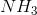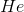None of these